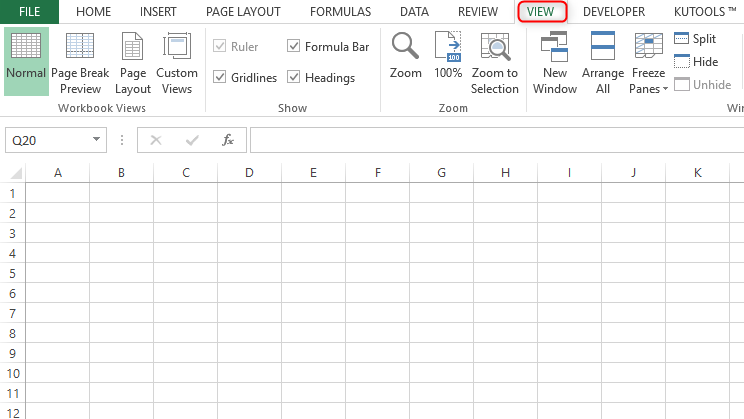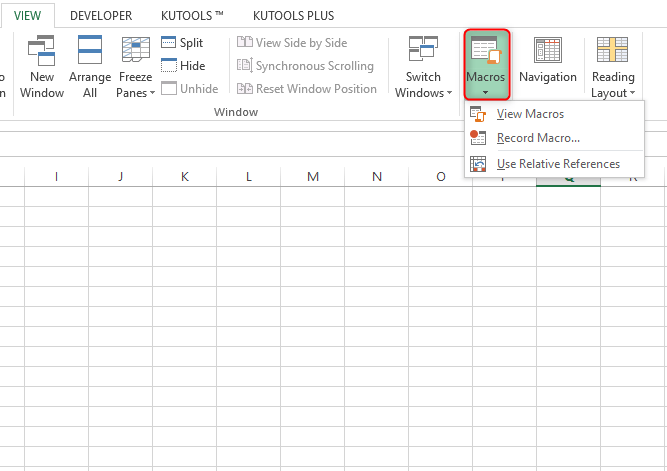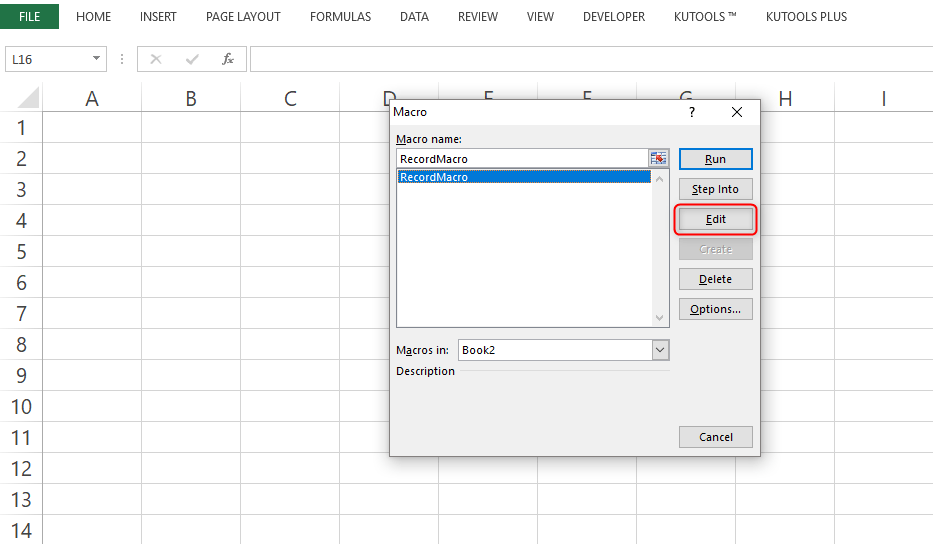# 10 Useful Examples of Macros for Beginners

## What are Macros?

They are a series of commands used to automate a repeated task. This can be run whenever the task must be performed.

### How to access Macros

Click on the 'View' tabIn the end, you'll find the function 'Macros' arranged in the Macros group. Click the arrow under 'Macros' where you can manage your macro performances easily.To edit a macro, click on the 'Edit' button, this will take you to the 'Visual Basic Editor' where you can easily modify the macro to do what you want.Visual Basic Editor view:## 10 Useful Examples of Macros for Accounting:

1. Macro: Save All

Helpful for saving all open excel workbooks at once. It is advised to run this macro before running other macros or performing tasks that could potentially freeze Microsoft Excel.

Name this macro 'SaveAll'

Example code:

 ``````Sub SaveAll() `````` ``````Application.ScreenUpdating = False `````` ``````Dim Wkb As Workbook `````` ``````For Each Wkb In Workbooks `````` ``````If Not Wkb.ReadOnly And Windows(Wkb.Name).Visible Then `````` ``````Wkb.Save `````` ``````End If `````` ``````Next `````` ``````Application.ScreenUpdating = True `````` ``End Sub``

It is helpful if you use a lot of comments and highlights while editing worksheets. Running this macro generates a new tab at the front of the worksheet with a listing of every cell with a comment or highlight in the workbook. Each cell reference is a hyperlink that leads directly to the cell with the comment/highlight. The summary tab lists the value within the cell and the text in the comment.

Additionally, there is an 'Accept' button that removes the highlight or deletes the comment from the chosen cell. Once all changes are accepted, the summary tab will be deleted.

NB: This macro is only set up to find certain highlights of yellow, but this can be modified.

3. Macro: Insert a Check Mark

Helpful for footing (Adding a column of numbers) trial balances, schedules, and reconciliations. It is often common to put a checkmark below the total to show the column total is accurate.

This macro will insert a red checkmark in the active cell. Once this is added all you will be required to do is select the cell you want to check-mark in and run the macro. Name this macro 'Checkmark'.

Example code:

 ``````Sub Checkmark() `````` ``````ActiveCell.FormulaR1C1 = "P" `````` ``````ActiveCell.Select `````` ``````With Selection.Font `````` ``````.Name = "Wingdings 2" `````` ``````.Size = 11 `````` ``````.Strikethrough = False `````` ``````.Superscript = False `````` ``````.OutlineFont = False `````` ``````.Shadow = False `````` ``````.Underline = xUnderlineStyleNone `````` ``````.ThemeColor = xThemeColorLight1 `````` ``````.TintAndShade = 0 `````` ``````.ThemeFont = xThemeFontNone `````` ``````End With `````` ``````Selection.Font.Bold = True `````` ``````With Selection.Font `````` ``````.Color = 16776961 `````` ``````.TintAndShade = 0 `````` ``````End With `````` ``````With Selection `````` ``````.HorizontalAlignment =xlCenter `````` ``````.VerticalAlignment = xlBottom `````` ``````.WrapText = False `````` ``````.Orientation = 0 `````` ``````.AddIndent = False `````` ``````.IndentLevel = 0 `````` ``````.ShrinkToFit = False `````` ``````.ReadingOrder = xlContent `````` ``````.MergeCells = False `````` ``````End With `````` ``End Sub``

4. Macro: Mail Workbook

Helpful to send a lot of excel files via email. Running this macro creates a new Microsoft Outlook email with the last saved version of the open workbook. The email, by default, will not be addressed by anyone, the subject will be the name of the workbook, and the body will be 'See attached'. However, these settings can be customized.

Example code:

 ``````Sub Mail_Workbook() `````` ``````Dim OutApp As Object `````` ``````Dim OutMail As Object `````` ``````Set OutApp = CreateObject("Outlook.Application") `````` ``````Set OutMail = OutApp.CreateItem(0) `````` ``````On Error Resume Next `````` ``````With OutMail `````` ``````.To = "" `````` ``````.CC = "" `````` ``````.BCC = "" `````` ``````.Subject = ActiveWorkbook.Name `````` ``````.body = "See attached." `````` ``````.Attachements.Add ActiveWorkbook.FullName `````` ``````.Display `````` ``````End With `````` ``````On Error GoTo 0 `````` ``````Set OutMail = Nothing `````` ``````Set OutApp = Nothing `````` ``End Sub``

5. Macro: Copying the Sum of Selected Cells

Helpful in copying the sum of several cells in a spreadsheet. These cells can be scattered throughout the spreadsheet or all in the same row/column.

Once this macro is added you need to highlight the cells you want to sum, then run the macro, and then paste it into the cell you want the sum in.

NB: This will just paste the value of the sum, not a sum() formula

Example code:

 ``````Sub CopySelectedSumValue() `````` ``````Dim MyDataObj As New DataObject `````` ``````MyDataObj.SetText Application.Sum(Selection) `````` ``````My DataObj.PutInClipboard `````` ``End Sub``

6. Macro: Open Calculator

Example code:

 Sub OpenCalculator() Application.ActivateMicrosoftApp Index:=0 End Sub

7. Macro: Refresh All Pivot Tables

Helpful to refresh all pivot tables in the whole workbook in a single shot.

Example code:

 ``````Sub RefreshAllPivotTables() `````` ``````Dim ws As Worksheet `````` ``````Dim pt As PivotTable `````` ``````For Each ws In ThisWorkbook.Worksheets `````` ``````For Each pt In ws.PivotTables `````` ``````pt.RefreshTable `````` ``````Next pt `````` ``````Next ws `````` ``End Sub``

8. Macro: Multiply all the Values by a Number

Helpful if you have a list of numbers you want to have multiplied by a particular number. Select the range of cells you need and run the example code below. It will first ask you for the number with whom you want to multiply and then instantly multiply all the numbers in the range with it.

Example code:

 ``````Sub multiplyWithNumber() `````` ``````Dim rng As Range `````` ``````Dim c As Integer c = InputBox("Enter number to multiple", "Input Required") `````` ``````For Each rng In Selection `````` ``````If WorksheetFunction.IsNumber(rng) Then `````` ``````rng.Value = rng * c `````` ``````Else `````` ``````End If `````` ``````Next rng `````` ``End Sub``

9. Macro: Add a Number to all the Numbers in Range

Similar to multiplying, you can also add a number to all the numbers in a particular range.

Example code:

 ``````Sub addNumber() `````` ``````Dim rngAs Range `````` ``````DimiAs Integer `````` ``````i= InputBox("Enter number to multiple", "Input Required") `````` ``````For Each rng In Selection `````` ``````If WorksheetFunction.IsNumber(rng) Then `````` ``````rng.Value= rng+ i `````` ``````Else `````` ``````End If `````` ``````Next rng `````` ``End Sub``

10. Macro: Remove Negative Signs

Code that checks a selection and converts all the negative numbers into positive. Select a range and run the code.

Example code:

 ``````Sub removeNegativeSign() `````` ``````Dim rngAs Range `````` ``````Selection.Value= Selection.Value `````` ``````For Each rngIn Selection `````` ``````If WorksheetFunction.IsNumber(rng) `````` ``````Then rng.Value= Abs(rng) `````` ``````End If `````` ``````Next rng `````` ``End Sub``